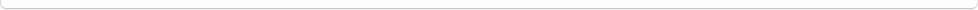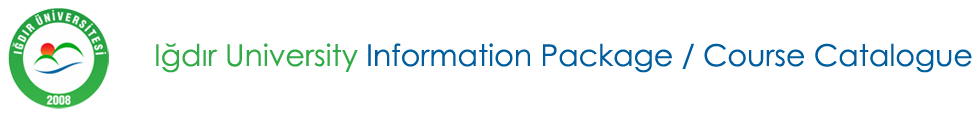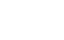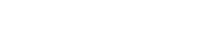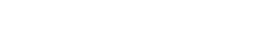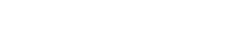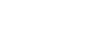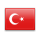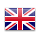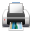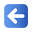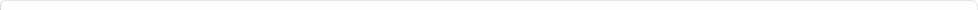Description of Individual Course Units
 Course Unit Code Course Unit Title Type of Course Unit Year of Study Semester Number of ECTS Credits 190500206100 APPLIED STATISTICAL TECHNIQUES IN COMMUNICATION SCIENCES Compulsory 3 6 4
Level of Course Unit
First Cycle
Objectives of the Course
With this course the student data collection, conversion to serial data and range of variability measures for calculation of probability calculation, to analyze the random variable, making sampling, application testing types, to analyze the relationship between variable and index calculations are intended to be trained in the subject.
Name of Lecturer(s)
Öğr. Gör. Mehmet POLAT
Learning Outcomes
 1 one 2 one 3 4
Mode of Delivery
Daytime Class
Prerequisites and co-requisities
None
Recommended Optional Programme Components
None
Course Contents
To collect the data, convert data to sequence and series of variability measures for calculation, calculate probabilities, to analyze the random variable, to sample, apply the test types, to analyze the relationship between the variables and make index calculations.
Weekly Detailed Course Contents
 Week Theoretical Practice Laboratory 1 To collect the data. 2 To convert the data series. 3 To convert the data series. 4 To calculate the dimensions of variability of the series. 5 To calculate the dimensions of variability of the series. 6 To calculate probabilities. 7 to analyze the random variable. 8 Midterm 9 sampling. 10 implement test types. 11 To analyze the relationship between variables. 12 To analyze the relationship between variables. 13 make index calculations. 14 Final exam
Textbook statistics, Ekrem Stars, EXCLUSIVE PUBLISHING, Basic Statistics course by Abdullah Thompson, Education Bookstore
Planned Learning Activities and Teaching Methods
Assessment Methods and Criteria
 Term (or Year) Learning Activities Quantity Weight Midterm Examination 1 100 SUM 100 End Of Term (or Year) Learning Activities Quantity Weight Final Examination 1 100 SUM 100 Term (or Year) Learning Activities 40 End Of Term (or Year) Learning Activities 60 SUM 100
Language of Instruction
Work Placement(s)
None